# 深入理解PHP传参原理

```PHP_FUNCTION(test)
{
char*  str;
int    str_len;

if (zend_parse_parameters(ZEND_NUM_ARGS() TSRMLS_CC, "s", &str, &str_len) == FAILURE) {
RETURN_FALSE;
}

php_printf(str);

// 无需free(str)
}```

`test("Hello World"); // 打印Hello World`

### EG(argument_stack)简介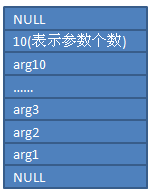EG(argument_stack)在php5.2中被具体实现为zend_ptr_stack类型:

```typedef struct _zend_ptr_stack {
int top;                       // 栈中当前元素的个数
int max;                       // 栈中最多存放元素的个数
void **elements;               // 栈底
void **top_element;            // 栈顶
} zend_ptr_stack;```

### 初始化argument_stack

```zend_ptr_stack_init(&EG(argument_stack));
zend_ptr_stack_push(&EG(argument_stack), (void *) NULL);```

zend_ptr_stack_init实现很简单。

```ZEND_API void zend_ptr_stack_init(zend_ptr_stack *stack)
{
stack->top_element = stack->elements = (void **) emalloc(sizeof(void *)*PTR_STACK_BLOCK_SIZE);
stack->max = PTR_STACK_BLOCK_SIZE;   // 栈的大小被初始化成64
stack->top = 0;                      // 当前元素个数为0
}```

NULL入栈之后，整个argument_stack的实际内存分布如下：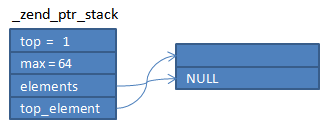### 参数入栈

```stack->top++;
*(stack->top_element++) = 参数;```

```function foo( \$str ){
print_r(123);
}
foo("hello world");```

 `line     # *  op                           fetch          ext  return  operands` `---------------------------------------------------------------------------------` `   ``3     0  >   NOP` `   ``6     1      SEND_VAL                                                  OP1[  IS_CONST (458754) 'hello world' ]` `         ``2      DO_FCALL                                      1           OP1[  IS_CONST (458752) 'foo' ]` `  ``15     3    > RETURN                                                    OP1[  IS_CONST (0) 1 ]`

SEND_VAL指令实际上做的事情就是将“hello world”压入argument_stack。

```int ZEND_SEND_VAL_SPEC_CONST_HANDLER(ZEND_OPCODE_HANDLER_ARGS)
{
……
zval *valptr, *value;

value = &opline->op1.u.constant;
ALLOC_ZVAL(valptr);
INIT_PZVAL_COPY(valptr, value);
if (!0) {
zval_copy_ctor(valptr);
}

// 入栈，valptr指向存放hello world的zval
zend_ptr_stack_push(&EG(argument_stack), valptr);
……
}```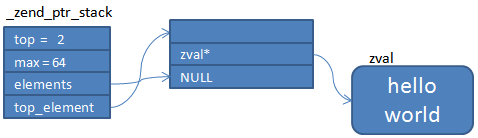### 参数个数

```static int zend_do_fcall_common_helper_SPEC(ZEND_OPCODE_HANDLER_ARGS)
{
……
// 在argument_stack中压入2个值
// 一个是参数个数（即opline->extended_value）
// 一个是标识栈顶的NULL
zend_ptr_stack_2_push(&EG(argument_stack), (void *)(zend_uintptr_t)opline->extended_value, NULL);
……
if (EX(function_state).function->type == ZEND_INTERNAL_FUNCTION) {
……
}
else if (EX(function_state).function->type == ZEND_USER_FUNCTION) {
……
// 调用foo函数
zend_execute(EG(active_op_array) TSRMLS_CC);
}
……
}
……
// 清理argument_stack
zend_ptr_stack_clear_multiple(TSRMLS_C);
……
ZEND_VM_NEXT_OPCODE();
}```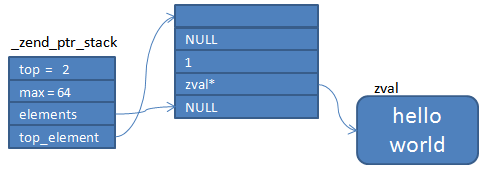### 获取参数

 `line     # *  op                           fetch          ext  return  operands` `---------------------------------------------------------------------------------` `   ``3     0  >   RECV                                                      OP1[  IS_CONST (0) 1 ]` `   ``4     1      SEND_VAL                                                  OP1[  IS_CONST (5) 123 ]` `         ``2      DO_FCALL                                      1           OP1[  IS_CONST (459027) 'print_r' ]` `   ``5     3    > RETURN                                                    OP1[  IS_CONST (0) null ]`

```static int ZEND_RECV_SPEC_HANDLER(ZEND_OPCODE_HANDLER_ARGS)
{
……// param拿参数的顺序是沿着栈顶-->栈底
if (zend_ptr_stack_get_arg(arg_num, (void **) &param TSRMLS_CC)==FAILURE) {
……
} else {
zend_free_op free_res;
zval **var_ptr;

// 验证参数
zend_verify_arg_type((zend_function *) EG(active_op_array), arg_num, *param TSRMLS_CC);
var_ptr = get_zval_ptr_ptr(&opline->result, EX(Ts), &free_res, BP_VAR_W);

// 获取参数
if (PZVAL_IS_REF(*param)) {
zend_assign_to_variable_reference(var_ptr, param TSRMLS_CC);
} else {
}
}

ZEND_VM_NEXT_OPCODE();
}```### 清理argument_stack

foo内部的print_r也是一个函数调用，因此也会产生压栈–>清栈的操作。因此print_r执行之前的argument_stack为：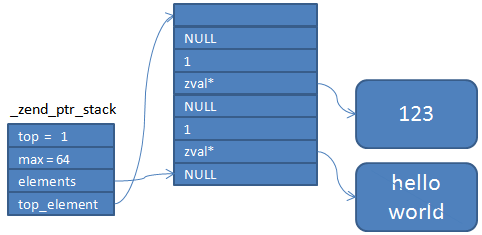print_r执行之后argument_stack又回到了foo刚RECV完的状态。

```static inline void zend_ptr_stack_clear_multiple(TSRMLS_D)
{
void **p = EG(argument_stack).top_element-2;
// 取栈顶保存的参数个数
int delete_count = (int)(zend_uintptr_t) *p;
EG(argument_stack).top -= (delete_count+2);

// 从上至下，依次清理
while (--delete_count>=0) {
zval *q = *(zval **)(--p);
*p = NULL;
zval_ptr_dtor(&q);
}
EG(argument_stack).top_element = p;
}```

 `value        "hello world"` `refcount     1` `type         6` `is_ref       0`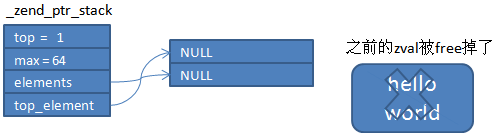### 回到文章刚开始的问题…

```PHP_FUNCTION(test)
{
char*  str;
int    str_len;

if (zend_parse_parameters(ZEND_NUM_ARGS() TSRMLS_CC, "s", &str, &str_len) == FAILURE) {
RETURN_FALSE;
}

str = 'H';
}```

```\$a = "hello world";
test(\$a);
echo \$a;```